### Home > CALC > Chapter 5 > Lesson 5.3.3 > Problem5-137

5-137.
1. Multiple Choice: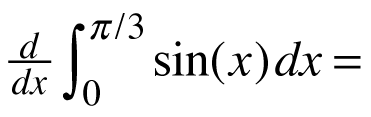Homework Help ✎

1.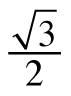2.3. 0

4.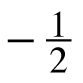5. undefined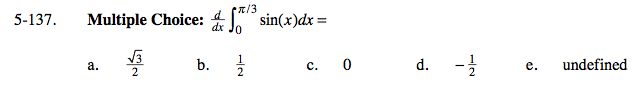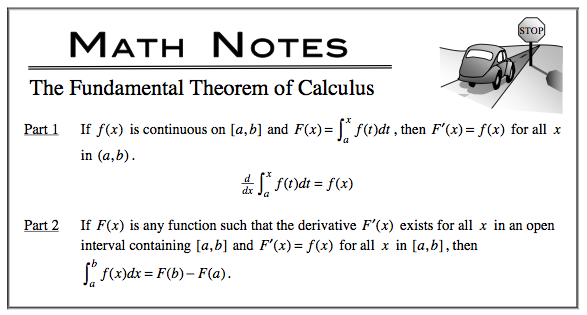Notice that the Fundamental Theorem of Calculus, Part 1 states that the derivative of an INDEFINITE integral is the original function.
This, however, is a DEFINITE integral, and a definite integral has a finite sum. What is the derivative of a finite value?